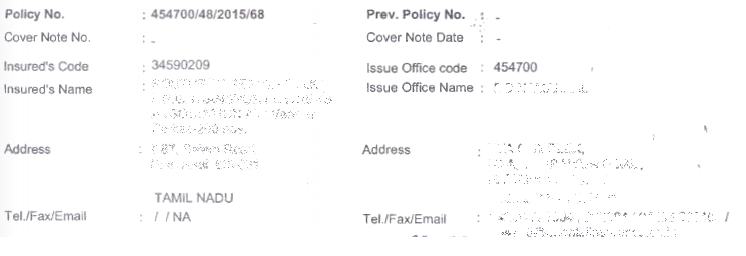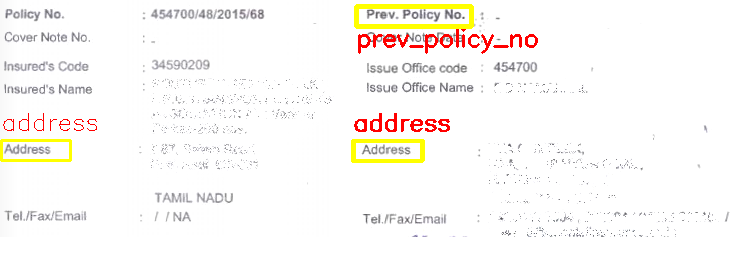# Python | Document field detection using Template Matching

Template matching is an image processing technique which is used to find the location of small-parts/template of a large image. This technique is widely used for object detection projects, like product quality, vahicle tracking, robotics etc.

In this article, we will learn how to use template matching for detecting the related fields in a document image.

Solution:
Above task can be achieved using template matching. Clip out the field images and apply template matching using clipped field images and the document image. The algorithm is simple yet reproducible into complex versions to solve the problem of field detection and localization for document images belonging to specific domains.

Approach:

• Clip/Crop field images from the main document and use them as separate templates.
• Define/tune thresholds for different fields.
• Apply template matching for each cropped field template using OpenCV function `cv2.matchTemplate()`
• Draw bounding boxes using the coordinates of rectangles fetched from template matching.
• Optional: Augment field templates and fine tune threshold to improve result for different document images.

Input Image:Output Image:Below is the Python code:

 `# importing libraries ` `import` `numpy as np ` `import` `imutils ` `import` `cv2 ` ` `  `field_threshold ``=` `{ ``"prev_policy_no"` `: ``0.7``, ` `                    ``"address"`        `: ``0.6``, ` `                  ``} ` ` `  `# Function to Generate bounding ` `# boxes around detected fields ` `def` `getBoxed(img, img_gray, template, field_name ``=` `"policy_no"``): ` ` `  `    ``w, h ``=` `template.shape[::``-``1``]  ` ` `  `    ``# Apply template matching ` `    ``res ``=` `cv2.matchTemplate(img_gray, template, ` `                           ``cv2.TM_CCOEFF_NORMED) ` ` `  `    ``hits ``=` `np.where(res >``=` `field_threshold[field_name]) ` ` `  `    ``# Draw a rectangle around the matched region.  ` `    ``for` `pt ``in` `zip``(``*``hits[::``-``1``]):  ` `        ``cv2.rectangle(img, pt, (pt[``0``] ``+` `w, pt[``1``] ``+` `h), ` `                                    ``(``0``, ``255``, ``255``), ``2``) ` ` `  `        ``y ``=` `pt[``1``] ``-` `10` `if` `pt[``1``] ``-` `10` `> ``10` `else` `pt[``1``] ``+` `h ``+` `20` ` `  `        ``cv2.putText(img, field_name, (pt[``0``], y), ` `            ``cv2.FONT_HERSHEY_SIMPLEX, ``0.8``, (``0``, ``0``, ``255``), ``1``) ` ` `  `    ``return` `img ` ` `  ` `  `# Driver Function ` `if` `__name__ ``=``=` `'__main__'``: ` ` `  `    ``# Read the original document image ` `    ``img ``=` `cv2.imread(``'doc.png'``) ` `       `  `    ``# 3-d to 2-d conversion ` `    ``img_gray ``=` `cv2.cvtColor(img, cv2.COLOR_BGR2GRAY) ` `      `  `    ``# Field templates ` `    ``template_add ``=` `cv2.imread(``'doc_address.png'``, ``0``) ` `    ``template_prev ``=` `cv2.imread(``'doc_prev_policy.png'``, ``0``) ` ` `  `    ``img ``=` `getBoxed(img.copy(), img_gray.copy(), ` `                       ``template_add, ``'address'``) ` ` `  `    ``img ``=` `getBoxed(img.copy(), img_gray.copy(), ` `                   ``template_prev, ``'prev_policy_no'``) ` ` `  `    ``cv2.imshow(``'Detected'``, img) `

• Computationally inexpensive.
• Easy to use and modifiable for different use-cases.
• Gives good results in case of document data scarcity.

• Result are not highly accurate as compared to segmentation techniques using deep learning.
• Lacks overlapping pattern problem resolution.

My Personal Notes arrow_drop_upCheck out this Author's contributed articles.

If you like GeeksforGeeks and would like to contribute, you can also write an article using contribute.geeksforgeeks.org or mail your article to contribute@geeksforgeeks.org. See your article appearing on the GeeksforGeeks main page and help other Geeks.

Please Improve this article if you find anything incorrect by clicking on the "Improve Article" button below.

Article Tags :

1

Please write to us at contribute@geeksforgeeks.org to report any issue with the above content.